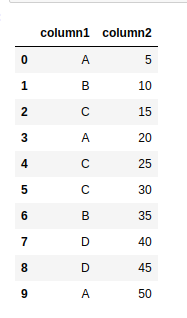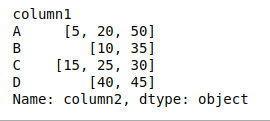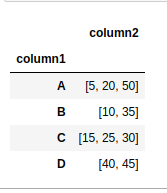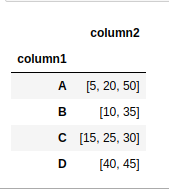# How to group dataframe rows into list in Pandas Groupby?

## How to group dataframe rows into list in Pandas Groupby?

Suppose you have a pandas DataFrame consisting of 2 columns and we want to group these columns. In this article, we will discuss about the same. First, let;s create the dataframe.

 `# importing pandas as pd` `import` `pandas as pd` ` ` ` ` `# Create the data frame` `df ``=` `pd.DataFrame({``'column1'``: [``'A'``, ``'B'``, ``'C'``, ``'A'``, ``'C'``,` `                               ``'C'``, ``'B'``, ``'D'``, ``'D'``, ``'A'``],` `                   ``'column2'``: [``5``, ``10``, ``15``, ``20``, ``25``, ``30``, ` `                             ``35``, ``40``, ``45``, ``50``]})` ` ` `# Print the dataframe` `df`

Output:Example #1: We can use groupby() method on column 1 and apply the method to apply a list on every group of pandas DataFrame.

 `# importing pandas as pd` `import` `pandas as pd` ` ` ` ` `# Create the data frame` `df ``=` `pd.DataFrame({``'column1'``: [``'A'``, ``'B'``, ``'C'``, ``'A'``, ``'C'``,` `                               ``'C'``, ``'B'``, ``'D'``, ``'D'``, ``'A'``],` `                   ``'column2'``: [``5``, ``10``, ``15``, ``20``, ``25``, ``30``, ` `                             ``35``, ``40``, ``45``, ``50``]})` ` ` ` ` `# Use groupby method and apply` `# method on the dataframe` `df ``=` `df.groupby(``'column1'``)[``'column2'``].``apply``(``list``)` ` ` `# Print the dataframe again` `df`

Output:Example #2: We can use groupby() method on column 1 and agg() method to apply aggregation, consisting of the lambda function, on every group of pandas DataFrame.

 `# importing pandas as pd` `import` `pandas as pd` ` ` ` ` `# Create the dataframe` `df ``=` `pd.DataFrame({``'column1'``: [``'A'``, ``'B'``, ``'C'``, ``'A'``, ``'C'``, ` `                               ``'C'``, ``'B'``, ``'D'``, ``'D'``, ``'A'``], ` `                   ``'column2'``: [``5``, ``10``, ``15``, ``20``, ``25``, ``30``,` `                               ``35``, ``40``, ``45``, ``50``]})` ` ` `# Use groupby method and agg method ` `# with lambda function on the dataframe` `df ``=` `df.groupby(``'column1'``).agg({``'column2'``: ``lambda` `x: ``list``(x)})` ` ` `# Print the dataframe again` `df`

Output:Example #3: We can use the groupby() method on column 1 and agg() method to apply the aggregation list, on every group of pandas DataFrame.

 `# importing pandas as pd` `import` `pandas as pd` ` ` ` ` `# Create the data frame` `df ``=` `pd.DataFrame({``'column1'``: [``'A'``, ``'B'``, ``'C'``, ``'A'``, ``'C'``,` `                               ``'C'``, ``'B'``, ``'D'``, ``'D'``, ``'A'``],` `                   ``'column2'``: [``5``, ``10``, ``15``, ``20``, ``25``, ``30``, ` `                               ``35``, ``40``, ``45``, ``50``]})` ` ` `# Use groupby method and agg method ` `# with list as argument on the dataframe` `df ``=` `df.groupby(``'column1'``).agg(``list``)` ` ` `df`

Output:Example #4: We can use groupby() method on column 1 and agg() method by passing ‘pd.Series.tolist’ as an argument.

 `# importing pandas as pd` `import` `pandas as pd` ` ` ` ` `# Create the data frame` `df ``=` `pd.DataFrame({``'column1'``: [``'A'``, ``'B'``, ``'C'``, ``'A'``, ``'C'``, ` `                               ``'C'``, ``'B'``, ``'D'``, ``'D'``, ``'A'``], ` `                   ``'column2'``: [``5``, ``10``, ``15``, ``20``, ``25``, ``30``,` `                               ``35``, ``40``, ``45``, ``50``]})` ` ` `# Use groupby method and agg method with` `# pd.Series.tolist as argument on the dataframe` `df ``=` `df.groupby(``'column1'``).agg(pd.Series.tolist)` ` ` `df`

Output:Last Updated on October 23, 2021 by admin

## One Hot Encoding to treat Categorical data parametersOne Hot Encoding to treat Categorical data parameters

ML | One Hot Encoding to treat Categorical data parameters Sometimes in datasets, we encounter

## Pandas dataframe.corr()Pandas dataframe.corr()

Pandas dataframe.corr()   Pandas dataframe.corr() is used to find the pairwise correlation of all columns

## Search A pandas Column For A ValueSearch A pandas Column For A Value

Search A pandas Column For A Value In this article let’s discuss how to search

## Sort Correlation Matrix in PythonSort Correlation Matrix in Python

Sort Correlation Matrix in Python A correlation matrix investigates the dependence between multiple variables at

## Python | Pandas Dataframe.pop()Python | Pandas Dataframe.pop()

Python | Pandas Dataframe.pop() Python is a great language for doing data analysis, primarily because

## Replace NaN Values with Zeros in Pandas DataFrameReplace NaN Values with Zeros in Pandas DataFrame

Missing data in a Pandas DataFrame is commonly represented as NaN (Not a Number) values.

## Pandas Built-in Data Visualization | MLPandas Built-in Data Visualization | ML

Pandas Built-in Data Visualization | ML Data Visualization is the presentation of data in graphical

## Pandas DataFrame.transformPandas DataFrame.transform

Python | Pandas DataFrame.transform Pandas DataFrame is a two-dimensional size-mutable, potentially heterogeneous tabular data structure